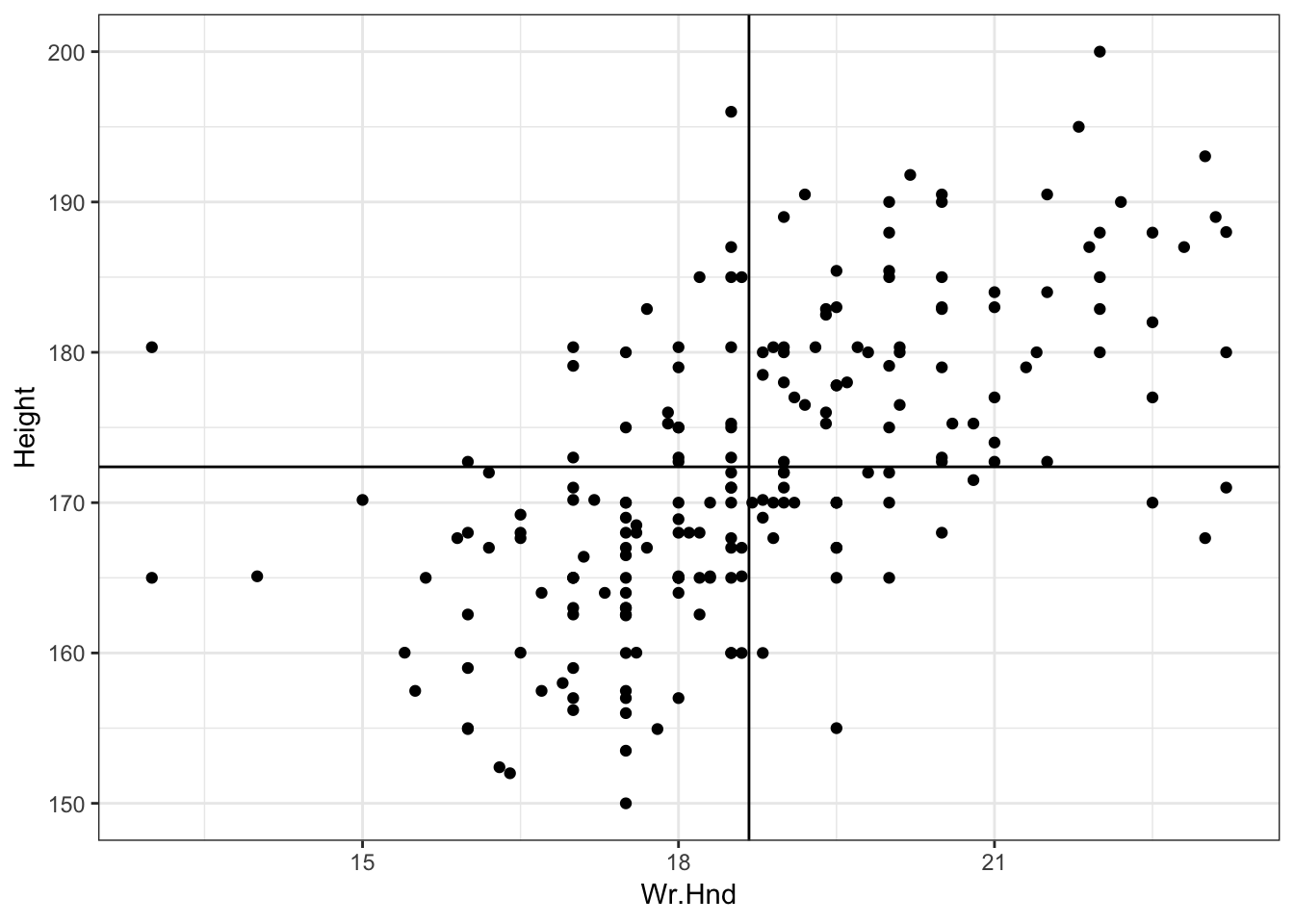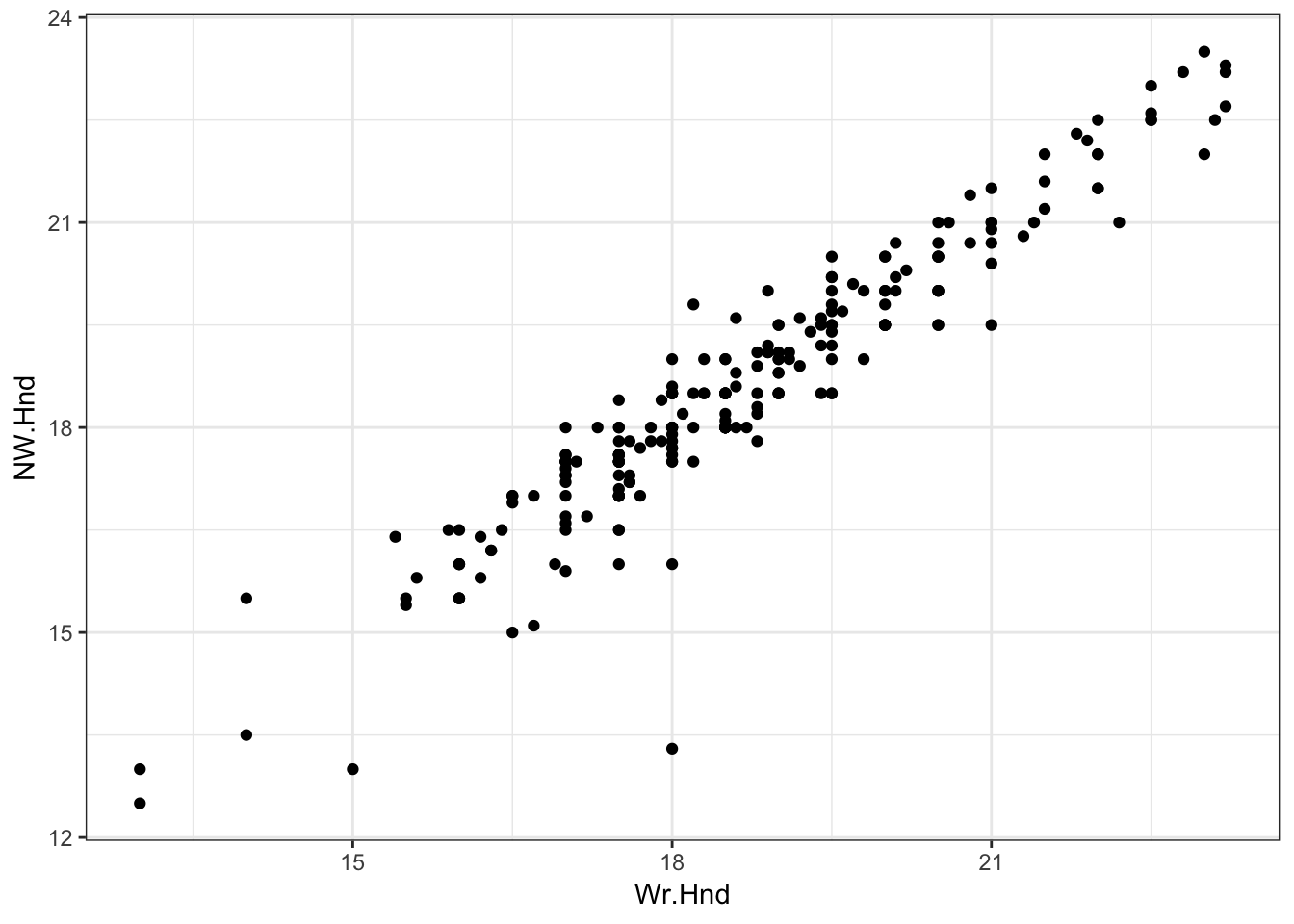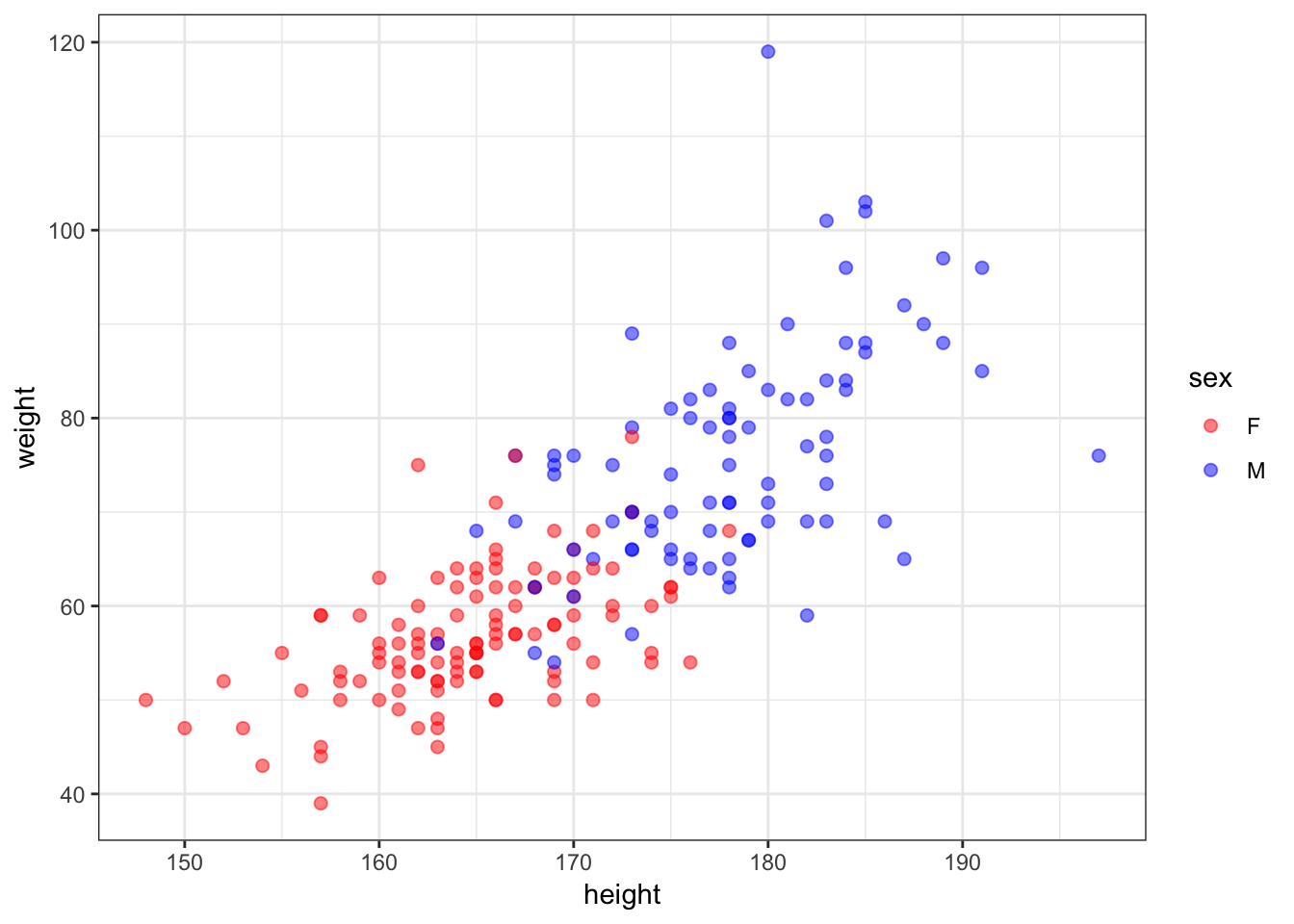55 Motivating Examples

55.1 Sample Correlation

Least squares regression “modelizes” correlation. Suppose we observe $$n$$ pairs of data $$(x_1, y_1), (x_2, y_2), \ldots, (x_n, y_n)$$. Their sample correlation is

$\begin{eqnarray} r_{xy} & = & \frac{\sum_{i=1}^n (x_i - \overline{x}) (y_i - \overline{y})}{\sqrt{\sum_{i=1}^n (x_i - \overline{x})^2 \sum_{i=1}^n (y_i - \overline{y})^2}} \\ \ & = & \frac{\sum_{i=1}^n (x_i - \overline{x}) (y_i - \overline{y})}{(n-1) s_x s_y} \end{eqnarray}$

where $$s_x$$ and $$s_y$$ are the sample standard deviations of each measured variable.

55.2 Example: Hand Size Vs. Height

> library("MASS")
> data("survey", package="MASS")
Sex Wr.Hnd NW.Hnd W.Hnd    Fold Pulse    Clap Exer Smoke Height
1 Female   18.5   18.0 Right  R on L    92    Left Some Never 173.00
2   Male   19.5   20.5  Left  R on L   104    Left None Regul 177.80
3   Male   18.0   13.3 Right  L on R    87 Neither None Occas     NA
4   Male   18.8   18.9 Right  R on L    NA Neither None Never 160.00
5   Male   20.0   20.0 Right Neither    35   Right Some Never 165.00
6 Female   18.0   17.7 Right  L on R    64   Right Some Never 172.72
M.I    Age
1   Metric 18.250
2 Imperial 17.583
3     <NA> 16.917
4   Metric 20.333
5   Metric 23.667
6 Imperial 21.000
> ggplot(data = survey, mapping=aes(x=Wr.Hnd, y=Height)) +
+   geom_point() + geom_vline(xintercept=mean(survey$Wr.Hnd, na.rm=TRUE)) + + geom_hline(yintercept=mean(survey$Height, na.rm=TRUE))55.3 Cor. of Hand Size and Height

> cor.test(x=survey$Wr.Hnd, y=survey$Height)

Pearson's product-moment correlation

data:  survey$Wr.Hnd and survey$Height
t = 10.792, df = 206, p-value < 2.2e-16
alternative hypothesis: true correlation is not equal to 0
95 percent confidence interval:
0.5063486 0.6813271
sample estimates:
cor
0.6009909

55.4 L/R Hand Sizes

> ggplot(data = survey) +
+   geom_point(aes(x=Wr.Hnd, y=NW.Hnd))55.5 Correlation of Hand Sizes

> cor.test(x=survey$Wr.Hnd, y=survey$NW.Hnd)

Pearson's product-moment correlation

data:  survey$Wr.Hnd and survey$NW.Hnd
t = 45.712, df = 234, p-value < 2.2e-16
alternative hypothesis: true correlation is not equal to 0
95 percent confidence interval:
0.9336780 0.9597816
sample estimates:
cor
0.9483103

55.6 Davis Data

> library("car")
> data("Davis", package="car")
> htwt <- tbl_df(Davis)
> htwt[12,c(2,3)] <- htwt[12,c(3,2)]
# A tibble: 6 x 5
sex   weight height repwt repht
<fct>  <int>  <int> <int> <int>
1 M         77    182    77   180
2 F         58    161    51   159
3 F         53    161    54   158
4 M         68    177    70   175
5 F         59    157    59   155
6 M         76    170    76   165

55.7 Height and Weight

> ggplot(htwt) +
+   geom_point(aes(x=height, y=weight, color=sex), size=2, alpha=0.5) +
+   scale_color_manual(values=c("red", "blue"))55.8 Correlation of Height and Weight

> cor.test(x=htwt$height, y=htwt$weight)

Pearson's product-moment correlation

data:  htwt$height and htwt$weight
t = 17.04, df = 198, p-value < 2.2e-16
alternative hypothesis: true correlation is not equal to 0
95 percent confidence interval:
0.7080838 0.8218898
sample estimates:
cor
0.7710743

55.9 Correlation Among Females

> htwt %>% filter(sex=="F") %>%
+   cor.test(~ height + weight, data = .)

Pearson's product-moment correlation

data:  height and weight
t = 6.2801, df = 110, p-value = 6.922e-09
alternative hypothesis: true correlation is not equal to 0
95 percent confidence interval:
0.3627531 0.6384268
sample estimates:
cor
0.5137293

55.10 Correlation Among Males

> htwt %>% filter(sex=="M") %>%
+   cor.test(~ height + weight, data = .)

Pearson's product-moment correlation

data:  height and weight
t = 5.9388, df = 86, p-value = 5.922e-08
alternative hypothesis: true correlation is not equal to 0
95 percent confidence interval:
0.3718488 0.6727460
sample estimates:
cor
0.5392906

Why are the stratified correlations lower?